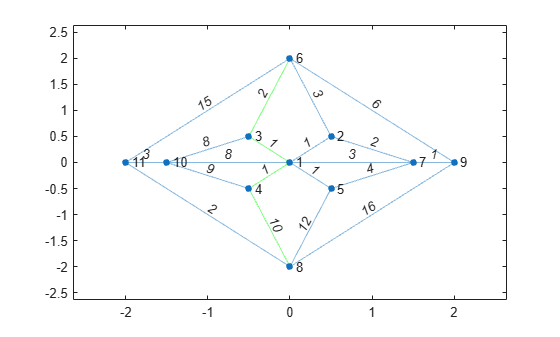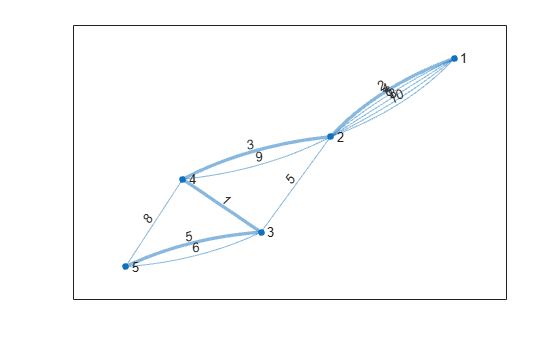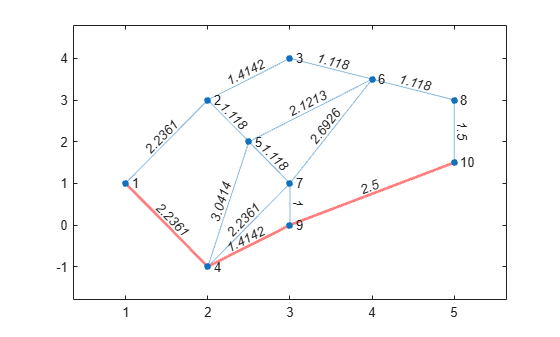shortestpath

Shortest path between two single nodes

Description

example

P = shortestpath(G,s,t) computes the shortest path starting at source node s and ending at target node t. If the graph is weighted (that is, G.Edges contains a variable Weight), then those weights are used as the distances along the edges in the graph. Otherwise, all edge distances are taken to be 1.

example

P = shortestpath(G,s,t,'Method',algorithm) optionally specifies the algorithm to use in computing the shortest path. For example, if G is a weighted graph, then shortestpath(G,s,t,'Method','unweighted') ignores the edge weights in G and instead treats all edge weights as 1.

example

[P,d] = shortestpath(___) additionally returns the length of the shortest path, d, using any of the input arguments in previous syntaxes.

example

[P,d,edgepath] = shortestpath(___) additionally returns the edge indices edgepath of all edges on the shortest path from s to t.

Examples

collapse all

Create and plot a directed graph.

s = [1 1 2 3 3 4 4 6 6 7 8 7 5];
t = [2 3 4 4 5 5 6 1 8 1 3 2 8];
G = digraph(s,t);
plot(G)Calculate the shortest path between nodes 7 and 8.

P = shortestpath(G,7,8)
P = 1×5

7     1     3     5     8

Create and plot a graph with weighted edges.

s = [1 1 1 2 2 6 6 7 7 3 3 9 9 4 4 11 11 8];
t = [2 3 4 5 6 7 8 5 8 9 10 5 10 11 12 10 12 12];
weights = [10 10 10 10 10 1 1 1 1 1 1 1 1 1 1 1 1 1];
G = graph(s,t,weights);
plot(G,'EdgeLabel',G.Edges.Weight)Find the shortest path between nodes 3 and 8, and specify two outputs to also return the length of the path.

[P,d] = shortestpath(G,3,8)
P = 1×5

3     9     5     7     8

d = 4

Since the edges in the center of the graph have large weights, the shortest path between nodes 3 and 8 goes around the boundary of the graph where the edge weights are smallest. This path has a total length of 4.

Create and plot a graph with weighted edges, using custom node coordinates.

s = [1 1 1 1 1 2 2 7 7 9 3 3 1 4 10 8 4 5 6 8];
t = [2 3 4 5 7 6 7 5 9 6 6 10 10 10 11 11 8 8 11 9];
weights = [1 1 1 1 3 3 2 4 1 6 2 8 8 9 3 2 10 12 15 16];
G = graph(s,t,weights);

x = [0 0.5 -0.5 -0.5 0.5 0 1.5 0 2 -1.5 -2];
y = [0 0.5 0.5 -0.5 -0.5 2 0 -2 0 0 0];
p = plot(G,'XData',x,'YData',y,'EdgeLabel',G.Edges.Weight);Find the shortest path between nodes 6 and 8 based on the graph edge weights. Highlight this path in green.

[path1,d] = shortestpath(G,6,8)
path1 = 1×5

6     3     1     4     8

d = 14
highlight(p,path1,'EdgeColor','g')Specify Method as unweighted to ignore the edge weights, instead treating all edges as if they had a weight of 1. This method produces a different path between the nodes, one that previously had too large of a path length to be the shortest path. Highlight this path in red.

[path2,d] = shortestpath(G,6,8,'Method','unweighted')
path2 = 1×3

6     9     8

d = 2
highlight(p,path2,'EdgeColor','r')Plot the shortest path between two nodes in a multigraph and highlight the specific edges that are traversed.

Create a weighted multigraph with five nodes. Several pairs of nodes have more than one edge between them. Plot the graph for reference.

G = graph([1 1 1 1 1 2 2 3 3 3 4 4],[2 2 2 2 2 3 4 4 5 5 5 2],[2 4 6 8 10 5 3 1 5 6 8 9]);
p = plot(G,'EdgeLabel',G.Edges.Weight);Find the shortest path between node 1 and node 5. Since several of the node pairs have more than one edge between them, specify three outputs to shortestpath to return the specific edges that the shortest path traverses.

[P,d,edgepath] = shortestpath(G,1,5)
P = 1×5

1     2     4     3     5

d = 11
edgepath = 1×4

1     7     9    10

The results indicate that the shortest path has a total length of 11 and follows the edges given by G.Edges(edgepath,:).

G.Edges(edgepath,:)
ans=4×2 table
EndNodes    Weight
________    ______

1    2       2
2    4       3
3    4       1
3    5       5

Highlight this edge path by using the highlight function with the 'Edges' name-value pair to specify the indices of the edges traversed.

highlight(p,'Edges',edgepath)Find the shortest path between nodes in a graph using the distance between the nodes as the edge weights.

Create a graph with 10 nodes.

s = [1 1 2 2 3 4 4 4 5 5 6 6 7 8 9];
t = [2 4 3 5 6 5 7 9 6 7 7 8 9 10 10];
G = graph(s,t);

Create x- and y-coordinates for the graph nodes. Then plot the graph using the node coordinates by specifying the 'XData' and 'YData' name-value pairs.

x = [1 2 3 2 2.5 4 3 5 3 5];
y = [1 3 4 -1 2 3.5 1 3 0 1.5];
plot(G,'XData',x,'YData',y)Add edge weights to the graph by computing the Euclidean distances between the graph nodes. The distance is calculated from the node coordinates $\left({\mathit{x}}_{\mathit{i}},{\mathit{y}}_{\mathit{i}}\right)$ as:

$\mathit{d}=\sqrt{{|\Delta \mathit{x}|}^{2}+{|\Delta \mathit{y}|}^{2}}=\sqrt{{|{\mathit{x}}_{\mathit{s}}-{\mathit{x}}_{\mathit{t}}|}^{2}+{|{\mathit{y}}_{\mathit{s}}-{\mathit{y}}_{\mathit{t}}|}^{2}}.$

To calculate $\Delta \mathit{x}$ and $\Delta \mathit{y}$, first use findedges to obtain vectors sn and tn describing the source and target nodes of each edge in the graph. Then use sn and tn to index into the x- and y-coordinate vectors and calculate $\Delta \mathit{x}={\mathit{x}}_{\mathit{s}}-{\mathit{x}}_{\mathit{t}}$ and $\Delta \mathit{y}={\mathit{y}}_{\mathit{s}}-{\mathit{y}}_{\mathit{t}}$. The hypot function computes the squareroot of the sum of squares, so specify $\Delta \mathit{x}$ and $\Delta \mathit{y}$ as the input arguments to calculate the length of each edge.

[sn,tn] = findedge(G);
dx = x(sn) - x(tn);
dy = y(sn) - y(tn);
D = hypot(dx,dy);

Add the distances to the graph as the edge weights and replot the graph with the edges labeled.

G.Edges.Weight = D';
p = plot(G,'XData',x,'YData',y,'EdgeLabel',G.Edges.Weight);Calculate the shortest path between node 1 and node 10 and specify two outputs to also return the path length. For weighted graphs, shortestpath automatically uses the 'positive' method which considers the edge weights.

[path,len] = shortestpath(G,1,10)
path = 1×4

1     4     9    10

len = 6.1503

Use the highlight function to display the path in the plot.

highlight(p,path,'EdgeColor','r','LineWidth',2)Input Arguments

collapse all

Input graph, specified as either a graph or digraph object. Use graph to create an undirected graph or digraph to create a directed graph.

Example: G = graph(1,2)

Example: G = digraph([1 2],[2 3])

Source and target node IDs, specified as separate arguments of node indices or node names.

ValueExample
Scalar node index1
Character vector node name'A'
String scalar node name"A"

Example: shortestpath(G,2,5) computes the shortest path between node 2 and node 5.

Example: shortestpath(G,'node1','node2') computes the shortest path between the named nodes node1 and node2.

Shortest path algorithm, specified as one of the options in the table.

OptionDescription
'auto' (default)

The 'auto' option automatically selects the algorithm:

• 'unweighted' is used for graph and digraph inputs with no edge weights.

• 'positive' is used for all graph inputs that have edge weights, and requires the weights to be nonnegative. This option is also used for digraph inputs with nonnegative edge weights.

• 'mixed' is used for digraph inputs whose edge weights contain some negative values. The graph cannot have negative cycles.

'unweighted'

Breadth-First computation that treats all edge weights as 1.

'positive'

Dijkstra algorithm that requires all edge weights to be nonnegative.

'mixed' (only for digraph)

Bellman-Ford algorithm for directed graphs that requires the graph to have no negative cycles.

While 'mixed' is slower than 'positive' for the same problem, 'mixed' is more versatile as it allows some edge weights to be negative.

'acyclic' (only for digraph)

Algorithm designed to improve performance for directed, acyclic graphs (DAGs) with weighted edges.

Use isdag to confirm if a directed graph is acyclic.

Note

For most graphs, 'unweighted' is the fastest algorithm, followed by 'acyclic', 'positive', and 'mixed'.

Example: shortestpath(G,s,t,'Method','acyclic')

Output Arguments

collapse all

Shortest path between nodes, returned as a vector of node indices or an array of node names. P is empty, {}, if there is no path between the nodes.

• If s and t contain numeric node indices, then P is a numeric vector of node indices.

• If s and t contain node names, then P is a cell array or string array containing node names.

If there are multiple shortest paths between s and t, then P contains only one of the paths. The path that is returned can change depending on which algorithm Method specifies.

Shortest path distance, returned as a numeric scalar. d is the summation of the edge weights between consecutive nodes in P. If there is no path between the nodes, then d is Inf.

Edges on shortest path, returned as a vector of edge indices. For multigraphs, this output indicates which edge between two nodes is on the path. This output is compatible with the 'Edges' name-value pair of highlight, for example: highlight(p,'Edges',edgepath).

Tips

• The shortestpath, shortestpathtree, and distances functions do not support undirected graphs with negative edge weights, or more generally any graph containing a negative cycle, for these reasons:

• A negative cycle is a path that leads from a node back to itself, with the sum of the edge weights on the path being negative. If a negative cycle is on a path between two nodes, then no shortest path exists between the nodes, since a shorter path can always be found by traversing the negative cycle.

• A single negative edge weight in an undirected graph creates a negative cycle.